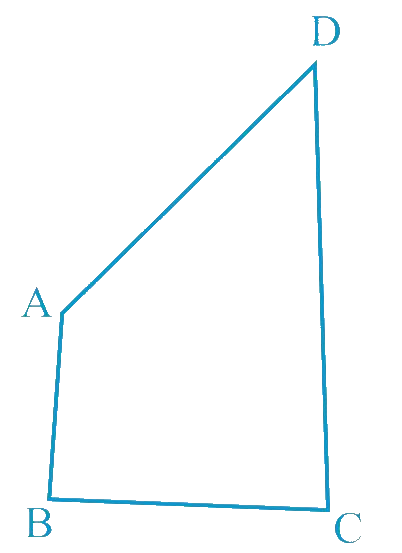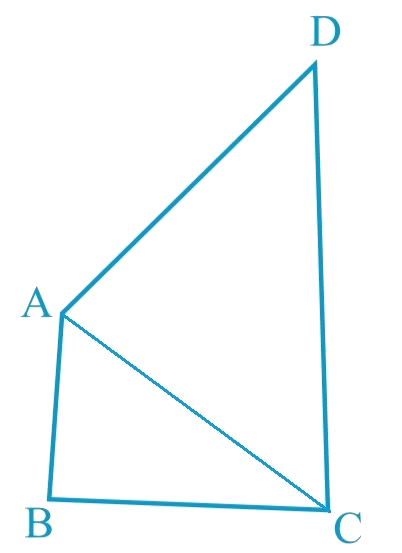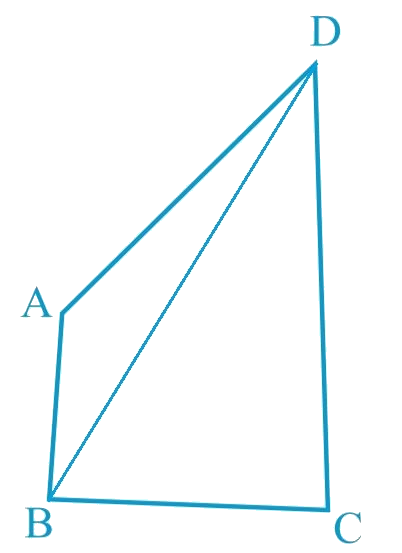# Ex.7.4 Q4 Triangles Solution - NCERT Maths Class 9

Go back to  'Ex.7.4'

## Question

$$AB$$ and $$CD$$ are respectively the smallest and longest sides of a quadrilateral $$ABCD$$ (see the given figure). Show that $$\angle A \gt \angle C$$ and $$\angle B \gt \angle D$$.

Video Solution
Triangles
Ex 7.4 | Question 4

## Text Solution

What is Known?

$$AB$$ and $$CD$$ are respectively the smallest and longest sides.

To prove:

$\angle \text{A}>\angle \text{C and }\angle \text{B}>\angle \text{D}$

Reasoning:

First of all we can join vertex A to C then triangles $$ABC$$ and $$ADC$$ will be formed now we can use the fact that In any triangle, the side opposite to the larger (greater) angle is longer We can add both the triangles result to get the first required result, Similarly we can join vertex B and D and use the fact to get the other required result.Steps:

Let us join $$AC$$.In $$\Delta ABC$$,

\begin{align}&AB>BC\\&\left( \begin{array} & \text{AB is the largest side } \\ \text{of quadrilateral}\,\,ABCD \\ \end{array} \right)\\\\&\angle2>\angle1\\&\left( \begin{array} & \text{Angle opposite to the } \\ \text{smaller side is smaller} \\ \end{array} \right)...\left( \text{1} \right)\end{align}

In $$\Delta ADC$$,

\begin{align}&AB>BC\\&\left( \begin{array} & \text{CD is the largest side } \\ \text{of quadrilateral}\,\,ABCD \\ \end{array} \right)\\\\&\angle4>\angle3\\&\left( \begin{array} & \text{Angle opposite to the } \\ \text{smaller side is smaller} \\ \end{array} \right)...\left( \text{2} \right)\end{align}

On adding Equations ($$1$$) and ($$2$$), we obtain

\begin{align}& \angle 2 + \angle 4 \lt \angle 1 + \angle 3\\ & \angle C \lt \angle A \\ & \angle A \gt \angle C \end{align}

Let us join $$BD,$$In $$\Delta ABD$$,

\begin{align}&AB>AD\\&\left( \begin{array} & \text{AB is the largest side } \\ \text{of quadrilateral}\,\,ABCD \\ \end{array} \right)\\\\&\angle8>\angle5\\&\left( \begin{array} & \text{Angle opposite to the } \\ \text{smaller side is smaller} \\ \end{array} \right)...\left( \text{3} \right)\end{align}

In $$\Delta BDC$$,

\begin{align}&BC>CD\\&\left( \begin{array} & \text{CD is the largest side } \\ \text{of quadrilateral}\,\,ABCD \\ \end{array} \right)\\\\&\angle7>\angle6\\&\left( \begin{array} & \text{Angle opposite to the } \\ \text{smaller side is smaller} \\ \end{array} \right)...\left( \text{4} \right)\end{align}

On adding Equations ($$3$$) and ($$4$$), we obtain

\begin{align}& \angle 8 + \angle 7 \lt \angle 5 + \angle 6\\ & \angle D \lt \angle B \\ & \angle B \gt \angle D \text{ (Hence, proved)}\end{align}

Learn from the best math teachers and top your exams

• Live one on one classroom and doubt clearing
• Practice worksheets in and after class for conceptual clarity
• Personalized curriculum to keep up with school